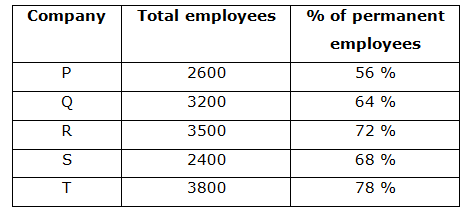# CWC/FCI Prelims 2019 – Quantitative Aptitude Questions (Day-18)

Dear Readers, Exam Race for the Year 2019 has already started, To enrich your preparation here we are providing new series of Practice Questions on Quantitative Aptitude – Section for CWC/FCI Exam. Aspirants, practice these questions on a regular basis to improve your score in aptitude section. Start your effective preparation from the right beginning to get success in upcoming CWC/FCI Exam.

[WpProQuiz 5592]

Directions (Q. 1 – 5): In the following questions, two equations I and II are given. You have to solve both the equations and give answer as,

a) If x > y

b) If x ≥ y

c) If x < y

d) If x ≤ y

e) If x = y or the relation cannot be established

1)

I) 5x – 3y = 0

II) 2x – 5y = – 19

2)

I) 3x2 – 447 = 420

II) y – √289 = 0

3)

I) 2x2 – 15x + 28 = 0

II) 3y2 – 13y + 14 = 0

4)

I) x2 – 18x + 77 = 0

II) y2 – 13y + 42 = 0

5)

I) x2 – 9x – 52 = 0

II) y2 – 7x – 44 = 0

Directions (Q. 6 – 10): Study the following information carefully and answer the given questions:

The following table shows the total number of employees and the percentage of permanent employees working in 6 different companies in a certain year.Note:

Total employees = Permanent employees + Temporary employees

6) Find the difference between the total number of temporary employees in Company P to that of total number of temporary employees in company T?

a) 452

b) 308

c) 626

d) 514

e) None of these

7) If the ratio between the male to that of female employees in company Q is 5: 3, then the ratio between the total number of male employees in company Q to that of total number of permanent employees in company P?

a) 237: 88

b) 52: 17

c) 77: 36

d) 125: 91

e) None of these

8) Find the average number of employees in all the given companies together?

a) 3100

b) 2800

c) 3250

d) 3600

e) None of these

9) Total number of permanent employees in company Q is approximately what percentage of total number of employees in company R?

a) 44 %

b) 67 %

c) 59 %

d) 75 %

e) 32 %

10) Total number of employees in company P and Q together is approximately what percentage more/less than the total number of employees in company S and T together?

a) 15 % more

b) 6 % less

c) 15 % less

d) 6 % more

e) 24 % less

Direction (1-5) :

5x – 3y = 0 –> (1)

2x – 5y = – 19 –> (2)

By solving the equation (1) and (2), we get,

X = 3, y = 5

X < y

I) 3x2 – 447 = 420

3x2 = 447 + 420

3x2 = 867

X2 = 289

X = 17, -17

II) y – √289 = 0

Y = √289

Y = 17

X ≤ y

I) 2x2 – 15x + 28 = 0

2x2 – 8x – 7x + 28 = 0

2x (x – 4) – 7 (x – 4) = 0

(2x – 7) (x – 4) = 0

X = 7/2, 4 = 3.5, 4

II) 3y2 – 13y + 14 = 0

3y2 – 6y – 7y + 14 = 0

3y (y – 2) – 7 (y – 2) = 0

(3y – 7) (y – 2) = 0

Y = 7/3, 2 = 2.33, 2

X > y

I) x2 – 18x + 77 = 0

(x – 11) (x – 7) = 0

X = 11, 7

II) y2 – 13y + 42 = 0

(y – 6) (y – 7) = 0

Y = 6, 7

X ≥ y

I) x2 – 9x – 52 = 0

(x – 13) (x + 4) = 0

X = 13, – 4

II) y2 – 7x – 44 = 0

(y – 11) (y + 4) = 0

Y = 11, -4

Can’t be determined

Direction (6-10) :

The total number of temporary employees in Company P

= > 2600*(44/100) = 1144

The total number of permanent employees in company T

= > 3800*(22/100) = 836

Required difference = 1144 – 836 = 308

Ratio between the male to that of female employees in company Q = 5: 3

The total number of male employees in company Q

= > 3200*(5/8) = 2000

The total number of permanent employees in company P

= > 2600*(56/100) = 1456

Required ratio = 2000: 1456 = 125: 91

The average number of employees in all the given companies together

= > (2600 + 3200 + 3500 + 2400 + 3800)/5

= > 15500/5 = 3100

Total number of permanent employees in company Q

= > 3200*(64/100) = 2048

Total number of employees in company R = 3500

Required % = (2048/3500)*100 = 58.514 % = 59 %

Total number of employees in company P and Q together

= > 2600 + 3200 = 5800

Total number of employees in company S and T together

= > 2400 + 3800 = 6200

Required % = [(6200 – 5800)/6200]*100 = 6.45 % = 6 % less